Chin. Phys. B, 2020, Vol. 29(11): 110505    DOI: 10.1088/1674-1056/abbbe3
 GENERAL Prev   Next

Dynamics of the two-SBT-memristor-based chaotic circuit

Mei Guo(郭梅), Meng Zhang(张萌), Ming-Long Dou(窦明龙), Gang Dou(窦刚), and Yu-Xia Li(李玉霞)
College of Electrical Engineering and Automation, Shandong University of Science and Technology, Qingdao 266590, China
 Abstract  A two-SBT-memristor-based chaotic circuit was proposed. The stability of the equilibrium point was studied by theoretical analysis. The close dependence of the circuit dynamic characteristics on its initial conditions and circuit parameters was investigated by utilizing Lyapunov exponents spectra, bifurcation diagrams, phase diagrams, and Poincaré maps. The analysis showed that the circuit system had complex dynamic behaviors, such as stable points, period, chaos, limit cycles, and so on. In particular, the chaotic circuit produced the multistability phenomenon, such as coexisting attractors and coexisting periods. Keywords:  memristor      chaotic circuit      multistability      coexisting attractors Received:  15 August 2020      Revised:  04 September 2020      Accepted manuscript online:  28 September 2020 Fund: the National Natural Science Foundation of China (Grant Nos. 61703247 and 61703246), the Qingdao Science and Technology Plan Project, China (Grant No. 19-6-2-2-cg), the Elite Project of Shandong University of Science and Technology, and the Taishan Scholar Project of Shandong Province of China. Corresponding Authors:  †Corresponding author. E-mail: dougang521@sdust.edu.cn ‡Corresponding author. E-mail: yuxiali2004@sdust.edu.cn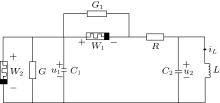Fig. 1.  The fifth-order chaotic circuit based on two SBT-memristors.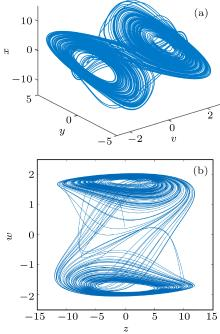Fig. 2.  The phase diagrams of the SBT-memristor-based circuit system.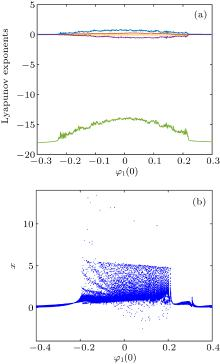Fig. 3.  Dynamics of the circuit with the change of initial conditions φ1(0): (a) Lyapunov exponent spectrum, and (b) bifurcation diagram of the state variable x.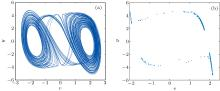Fig. 4.  (a) The phase diagram on the v–y plane, and (b)the corresponding Poincaré map when the initial conditions φ1(0) = −0.08.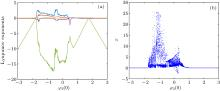Fig. 5.  Dynamics of the circuit with the change of initial conditions φ2(0): (a) Lyapunov exponent spectrum, and (b) bifurcation diagram of the state variable x.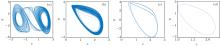Fig. 6.  The phase diagrams of the circuit with different initial conditions φ2(0) values: (a) φ2(0) = 0.1, (b) φ2(0) = 0.38, (c) φ2(0) = 0.4, and (d) φ2(0) = 0.65.Table 1.  The dynamics with variation in initial conditions φ2(0).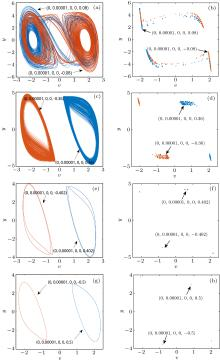Fig. 7.  The multistability of the circuit with different initial conditions φ2(0) values: (a) the phase diagram when φ2 (0) = ±0.08, (b) the Poincaré map when φ2 (0) = ±0.08, (c) the phase diagram when φ2 (0) = ±0.36, (d) the Poincaré map when φ2 (0) = ±0.36, (e) the phase diagram when φ2 (0) = ±0.402, (f) the Poincaré map when φ2 (0) = ±0.402, (g) the phase diagram when φ2 (0) = ±0.5, and (h) the Poincaré map when φ2 (0) = ±0.5.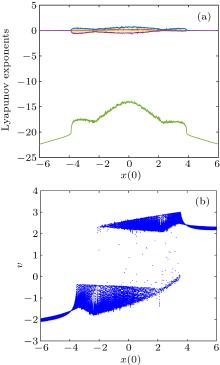Fig. 8.  Dynamics of the circuit with the change of initial conditions x(0): (a) Lyapunov exponent spectrum, and (b) bifurcation diagram of the state variable v.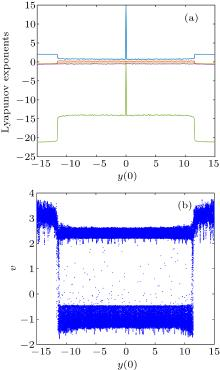Fig. 9.  Dynamics of the circuit with the change of initial conditions y(0): (a) Lyapunov exponent spectrum, and (b) bifurcation diagram of the state variable v.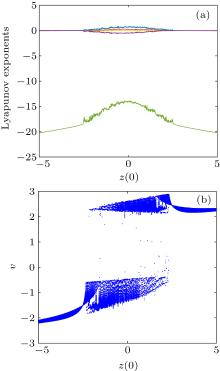Fig. 10.  Dynamics of the circuit with the change of initial conditions z(0): (a) Lyapunov exponent spectrum, and (b) bifurcation diagram of the state variable v.Table 2.  The dynamics with the variation in initial conditions x(0), y(0), and z(0).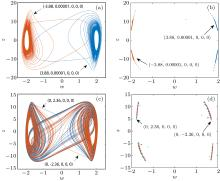Fig. 11.  The multistability of the circuit with different initial conditions values: (a) the phase diagram when x(0) = ±3.88, (b) the Poincaré map when x(0) = ±3.88, (c) the phase diagram when y(0) = ±2.36, and (d) the Poincaré map when y(0) = ±2.36.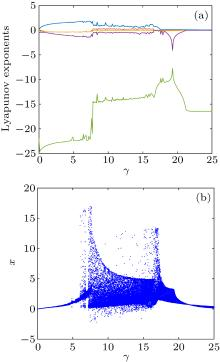Fig. 12.  Dynamics of the circuit with the change of circuit parameters γ: (a) Lyapunov exponent spectrum, and (b) bifurcation diagram of the state variable x.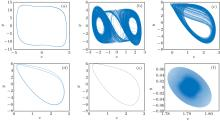Fig. 13.  The dynamical evolutions of the circuit with the change of the parameter γ: (a) γ = 4.29, (b) γ = 12.94, (c) γ = 17, (d) γ = 17.91, (e) γ = 18.6, and (f) γ = 23.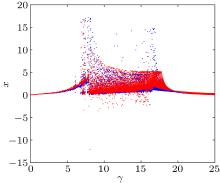Fig. 14.  The coexisting bifurcation diagram of the circuit parameter γ with the initial conditions (±1, 0.00001, 0, 0, 0).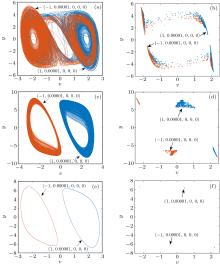Fig. 15.  The multistability of the circuit with different circuit parameter γ values: (a) the phase diagram when γ = 12.9, (b) the Poincaré map when γ = 12.9, (c) the phase diagram when γ = 17, (d) the Poincaré map when γ = 17, (e) the phase diagram when γ = 19.05, and (f) the Poincaré map when γ = 19.05.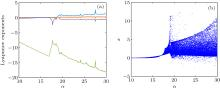Fig. 16.  Dynamics of the circuit with the change of circuit parameters α: (a) Lyapunov exponent spectrum, and (b) bifurcation diagram of the state variable x.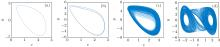Fig. 17.  The phase diagrams of the circuit with different circuit parameter α values: (a) α = 17.15, (b) γ = 18.51, (c) α = 19.1 and (d) α = 25.5.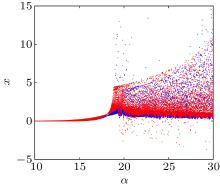Fig. 18.  The coexisting bifurcation diagram of the circuit parameter α with the initial conditions (±1, 0.00001, 0, 0, 0).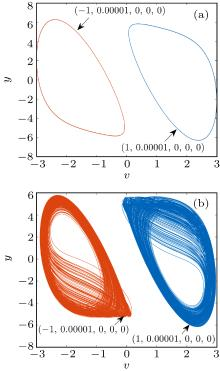Fig. 19.  The multistability of the circuit with different circuit parameter α values: (a) α = 18.65 and (b) α = 19.2.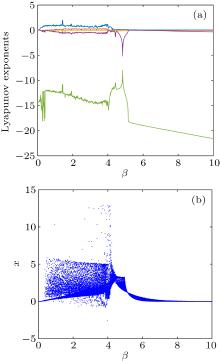Fig. 20.  Dynamics of the circuit with the change of circuit parameters β: (a) Lyapunov exponent spectrum, and (b) bifurcation diagram of the state variable x.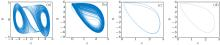Fig. 21.  The phase diagrams of the circuit with different circuit parameter β values: (a) β = 2.53, (b) β = 4.13, (c) β = 4.33, and (d) β = 4.45.Table 3.  The dynamics with the variation in circuit parameters α, β, and r.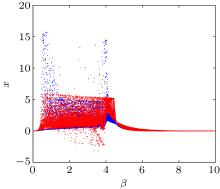Fig. 22.  The coexisting bifurcation diagram of the circuit parameter β with the initial conditions (±1, 0.00001, 0, 0, 0).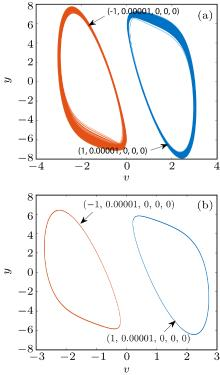Fig. 23.  The multistability of the circuit with different circuit parameter β values: (a) β = 4.11 and (b) β = 4.5.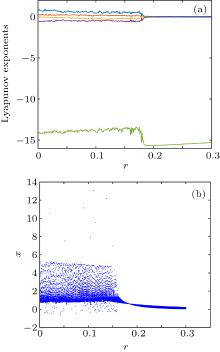Fig. 24.  Dynamics of the circuit with the change of circuit parameters r: (a) Lyapunov exponent spectrum, and (b) bifurcation diagram of the state variable x.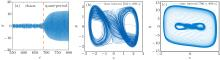Fig. 25.  The phenomenon of transient chaos when β = 0.59: (a) time-domain trajectory in the interval of [550 s, 800 s], (b) the phase diagram in time interval of [550 s, 680 s], and (c) the phase diagram in time interval of [700 s, 800 s].